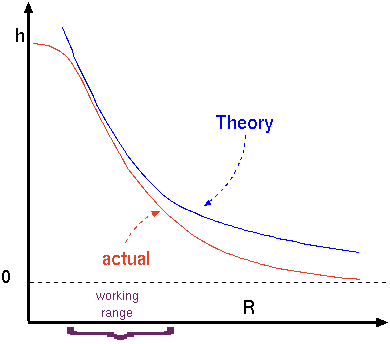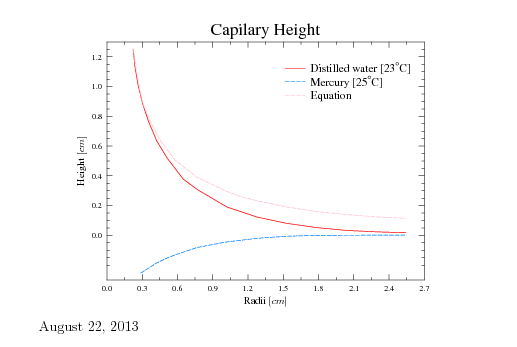# 1.7.1.1: Capillarity

The capillary forces referred to the fact that surface tension causes liquid to rise or penetrate into area (volume), otherwise it will not be there. It can be shown that the height that the liquid raised in a tube due to the surface tension is $h = \frac{2\sigma\cos(\beta)}{g\Delta\rho r}\tag{73}$ Where $$\Delta\rho$$ is the difference of liquid density to the gas density and $$r$$ is the radius of the tube.Fig. 1.24. The raising height as a function of the radii.

But this simplistic equation is unusable and useless unless the contact angle (assuming that the contact angel is constant or a repressive average can be found or provided or can be measured) is given. However, in reality there is no readily information for contact angle and therefore this equation is useful to show the treads. The maximum that the contact angle can be obtained in equation (73) when $$\beta$$ = - and thus $$\cos(\beta) = 1$$. This angle is obtained when a perfect half a sphere shape exist of the liquid surface. In that case equation (73) becomes $h_{max} = \frac{2\sigma}{g\Delta\rho r}\tag{74}$Fig. 1.25. The raising height as a function of the radius.

Figure 1.25 exhibits the height as a function of the radius of the tube. The height based on equation (74) is shown in Figure 1.24 as a blue line. The actual height is shown in the red line. Equation (74) provides reasonable results only in a certain range. for a small tube radius, equation (62) proved better results because the curve approaches hemispherical sphere(small gravity effect). For large radii equation (62) approaches the strait line (the liquid line) strong gravity effect. On the other hand, for extremely small radii equation (74) indicates that the high height which indicates a negative pressure. The liquid at a certain pressure will be vaporized and will breakdown the model upon this equation was constructed. Furthermore, the small scale indicates that the simplistic and continuous approach is not appropriate and a different model is needed. The conclusion of this discussion are shown in Figure 1.24. The actual dimension for many liquids (even water) is about 1-5 $$[mm]$$.

The discussion above was referred to "wetting'' contact angle. The depression of the liquid occurs in a "negative'' contact angle similarly to wetting.'' The depression height, $$h$$ is similar to equation (74) with a minus sign. However, the gravity is working against the surface tension and reducing the range and quality of the predictions of equation (74) The measurements of the height of distilled water and mercury are presented in Figure 1.25. The experimental results of these materials are with agreement with the discussion above. The surface tension of a selected material is given in Table 1.7.

In conclusion, the surface tension issue is important only in case where the radius is very small and gravity is negligible. The surface tension depends on the two materials or mediums that it separates.

Example 1.19

Calculate the diameter of a water droplet to attain pressure difference of 1000[$$N/m^2$$]. You can assume that temperature is $$20^{\circ}C$$.

Solution 1.19

The pressure inside the droplet is given by equation (50).

$D = 2\,R = \dfrac{2\,2\,\sigma}{\Delta P} = \dfrac{4\times 0.0728 }{1000} \sim 2.912\,{10}^{-4} [m] \tag{75}$

Example 1.20

Calculate the pressure difference between a droplet of water at $$20^{\circ}C$$ when the droplet has a diameter of 0.02 cm.

Solution 1.20

using equation
$\Delta P = \dfrac{2\,\sigma}{r} \sim \dfrac{2\times 0.0728}{0.0002} \sim 728.0 [N/m^2] \tag{76}$

Example 1.21

Calculate the maximum force necessary to lift a thin wire ring of 0.04[m] diameter from a water surface at $$20^{\circ}C$$ . Neglect the weight of the ring.

Solution 1.21

$F = 2(2\,\pi\,r\,\sigma)\cos\beta \tag{77}$
The actual force is unknown since the contact angle is unknown. However, the maximum Force is obtained when $$\beta = 0$$ and thus $$\cos\beta = 1$$. Therefore,
$F = 4\,\pi\,r\,\sigma = 4\times \pi \times 0.04\times 0.0728 \sim .0366 [N] \tag{78}$
In this value the gravity is not accounted for.

Example 1.22

A small liquid drop is surrounded with the air and has a diameter of 0.001 [m]. The pressure difference between the inside and outside droplet is 1[kPa]. Estimate the surface tension?

Solution 1.22

To be continue

 Chemical formula Temperature, $$T\,[^{\circ}C]$$ Surface Tension, $$\left[\dfrac{ N}{m} \right]$$ correction Acetic Acid 27.6 20 n/a Acetone 25.20 - -0.1120 Aniline 43.4 22 -0.1085 Benzene 28.88 - -0.1291 Benzylalcohol 39.00 - -0.0920 Benzylbenzoate 45.95 - -0.1066 Bromobenzene 36.50 - -0.1160 Bromobenzene 36.50 - -0.1160 Bromoform 41.50 - -0.1308 Butyronitrile 28.10 - -0.1037 Carbon disulfid 32.30 - -0.1484 Quinoline 43.12 - -0.1063 Chloro benzene 33.60 - -0.1191 Chloroform 27.50 - -0.1295 Cyclohexane 24.95 - -0.1211 Cyclohexanol 34.40 $$25^{\circ}C$$ -0.0966 Cyclopentanol 32.70 - -0.1011 Carbon Tetrachloride 26.8 - n/a Carbon disulfid 32.30 - -0.1484 Chlorobutane 23.10 - -0.1117 Ethyl Alcohol 22.3 - n/a Ethanol 22.10 - -0.0832 Ethylbenzene 29.20 - -0.1094 Ethylbromide 24.20 - -0.1159 Ethylene glycol 47.70 - -0.0890 Formamide 58.20 - -0.0842 Gasoline ∼ 21 - n/a Glycerol 64.0 - -0.0598 Helium 0.12 $$-269^{\circ}C$$ n/a Mercury 425-465.0 - -0.2049 Methanol 22.70 - -0.0773 Methyl naphthalene 38.60 - -0.1118 Methyl Alcohol 22.6 - n/a Neon 5.15 $$-247^{\circ}C$$ n/a Nitrobenzene 43.90 - -0.1177 Olive Oil 43.0-48.0 - -0.067 Perfluoroheptane 12.85 - -0.0972 Perfluorohexane 11.91 - -0.0935 Perfluorooctane 14.00 - -0.0902 Phenylisothiocyanate 41.50 - -0.1172 Propanol 23.70 $$25^{\circ}C$$ -0.0777 Pyridine 38.00 - -0.1372 Pyrrol 36.60 - -0.1100 SAE 30 Oil n/a - n/a Seawater 54-69 - n/a Toluene 28.4 - -0.1189 Turpentine 27 - n/a Water 72.80 - -0.1514 o-Xylene 30.10 - -0.1101 m-Xylene 28.90 - -0.1104

## Contributors

• Dr. Genick Bar-Meir. Permission is granted to copy, distribute and/or modify this document under the terms of the GNU Free Documentation License, Version 1.2 or later or Potto license.